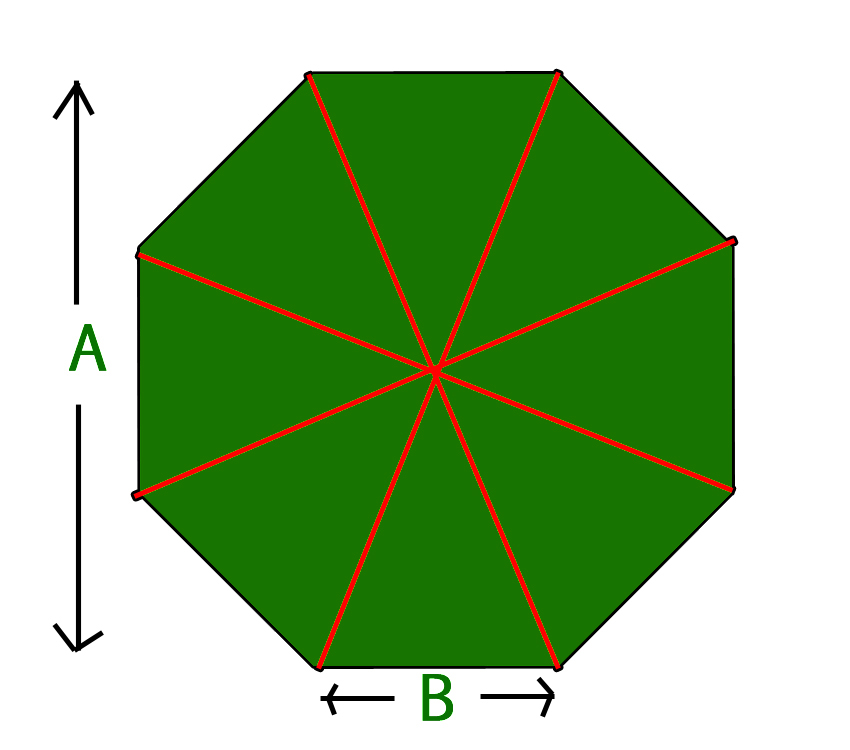Open in App
Not now

# Program to calculate Area Of Octagon

• Last Updated : 17 Feb, 2023

A regular octagon is a closed figure with sides of the same length and internal angles of the same size. It has eight lines of reflective symmetry and rotational symmetry of order 8. The internal angle at each vertex of a regular octagon is 135°. The central angle is 45°.
Properties :

Convex polygon, Equilateral polygon, Isogonal figure, Isotoxal figure, Cyclic.Formula :

`Area : 2 × (side length)² × (1+sqrt(2))`

Examples :

```Input : side = 3
Output : Area of Regular Octagon = 43.4558

Input : side = 4
Output : Area of Regular Octagon = 77.2548```

## C++

 `// CPP program to find area of octagon``#include ``using` `namespace` `std;`` ` `// Utility function``double` `areaOctagon(``double` `side)``{``    ``return` `(``float``)(2 * (1 + ``sqrt``(2)) * ``                   ``side * side);``}`` ` `// Driver Code``int` `main()``{``    ``double` `side = 4;``    ``cout << ``"Area of Regular Octagon = "``         ``<< areaOctagon(side) << endl;``    ``return` `0;``}`

## Java

 `// Java Program to find ``// area of Octagon.``import` `java.io.*;`` ` `class` `GFG``{   ``    ``// utility function``    ``static` `double` `areaOctagon(``double` `side)``    ``{``    ``return` `(``float``)(``2` `* (``1` `+ Math.sqrt(``2``)) ``                          ``* side * side);``    ``}``     ` `    ``// driver code``    ``public` `static` `void` `main(String arg[])``    ``{``        ``double` `side = ``4``;``        ``System.out.print(``"Area of Regular Octagon = "`  `                          ``+ areaOctagon(side));``    ``}``}`` ` `// This code is contributed by Anant Agarwal.`

## Python3

 `# Python3 program to ``# find area of octagon`` ` `import` `math`` ` `# Utility function``def` `areaOctagon(side):``    ``return` `(``2` `*` `(``1` `+` `(math.sqrt(``2``))) ``*` `side ``*` `side)`` ` `# Driver function``side ``=` `4``print``(``"Area of Regular Octagon ="``,``       ``round``(areaOctagon(side), ``4``))`` ` `# This code is contributed``# by Azkia Anam.`

## C#

 `// C# Program to find ``// area of Octagon.``using` `System;`` ` `class` `GFG``{ ``    ``// utility function``    ``static` `double` `areaOctagon(``double` `side)``    ``{``    ``return` `(``float``)(2 * (1 + Math.Sqrt(2)) ``                        ``* side * side);``    ``}``     ` `    ``// Driver code``    ``public` `static` `void` `Main()``    ``{``        ``double` `side = 4;``        ``Console.WriteLine(``"Area of Regular Octagon = "``                          ``+ areaOctagon(side));``    ``}``}`` ` `// This code is contributed by vt_m.`

## PHP

 ``

## Javascript

 ``

Output :

`Area of Regular Octagon = 77.25`

Time complexity : O(1)
Auxiliary Space : O(1)

My Personal Notes arrow_drop_up